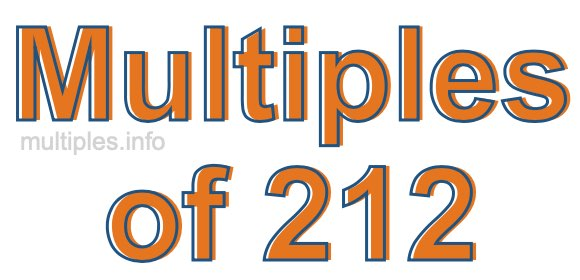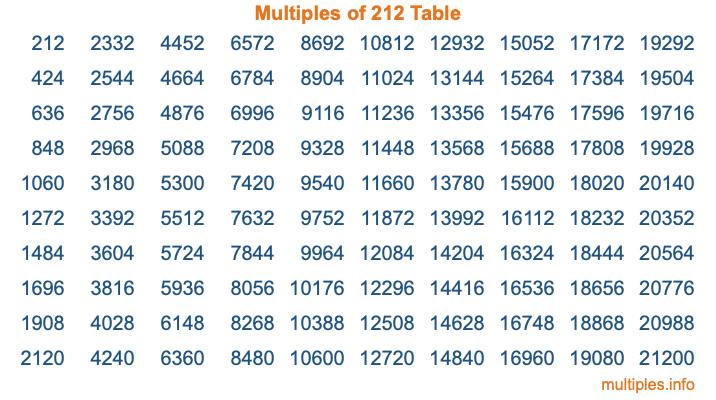Multiples of 212Welcome to the Multiples of 212 page. Here we will first teach you everything you will ever need to know about the multiples of 212, and then give you a study guide summary of everything we taught you to make sure you remember it all. Use this page to look up facts and learn information about the multiples of 212. This page will make you a multiples of two hundred twelve expert!

Definition of Multiples of 212
Multiples of 212 are all the numbers that when divided by 212 equal an integer. Each of the multiples of 212 are called a multiple. A multiple of 212 is created by multiplying 212 by an integer.

Therefore, to create a list of multiples of 212, you start with 1 multiplied by 212, then 2 multiplied by 212, then 3 multiplied by 212, and so on for as long as you want. Thus, the list of the first five multiples of 212 is 212, 424, 636, 848, and 1060. To see a larger list of multiples of 212, see the printable image of Multiples of 212 further down on this page. We also have a category where you can choose any nth multiple of 212.

Multiples of 212 Checker
The Multiples of 212 Checker below checks to see if any number of your choice is a multiple of 212. In other words, it checks to see if there is any number (integer) that when multiplied by 212 will equal your number. To do that, we divide your number by 212. If the the quotient is an integer, then your number is a multiple of 212.

Is  a multiple of 212?

Least Common Multiple of 212 and ...
A Least Common Multiple (LCM) is the lowest multiple that two or more numbers have in common. This is also called the smallest common multiple or lowest common multiple and is useful to know when you are adding our subtracting fractions. Enter one or more numbers below (212 is already entered) to find the LCM.

Check out our LCM Calculator if you need more details about the Least Common Multiple or if you need the LCM for different numbers for adding and subtraction fractions.

nth Multiple of 212
As we stated above, 212 is the first multiple of 212, 424 is the second multiple of 212, 636 is the third multiple of 212, and so on. Enter a number below to find the nth multiple of 212.

th multiple of 212

Multiples of 212 vs Factors of 212
212 is a multiple of 212 and a factor of 212, but that is where the similarities end. All postive multiples of 212 are 212 or greater than 212. All positive factors of 212 are 212 or less than 212.

Below is the beginning list of multiples of 212 and the factors of 212 so you can compare:

Multiples of 212: 212, 424, 636, 848, 1060, etc.

Factors of 212: 1, 2, 4, 53, 106, 212

As you can see, the multiples of 212 are all the numbers that you can divide by 212 to get a whole number. The factors of 212, on the other hand, are all the whole numbers that you can multiply by another whole number to get 212.

It's also interesting to note that if a number (x) is a factor of 212, then 212 will also be a multiple of that number (x).

Multiples of 212 vs Divisors of 212
The divisors of 212 are all the integers that 212 can be divided by evenly. Below is a list of the divisors of 212.

Divisors of 212: 1, 2, 4, 53, 106, 212

The interesting thing to note here is that if you take any multiple of 212 and divide it by a divisor of 212, you will see that the quotient is an integer.

Multiples of 212 Table
Below is an image of the first 100 multiples of 212 in a table. The table is in chronological order, column by column. The first column has the first ten multiples of 212, the second column has the next ten multiples of 212, and so on.The Multiples of 212 Table is also referred to as the 212 Times Table or Times Table of 212. You are welcome to print out our table for your studies.

Negative Multiples of 212
Although not often discussed or needed in math, it is worth mentioning that you can make a list of negative multiples of 212 by multiplying 212 by -1, then by -2, then by -3, and so on, to get the following list of negative multiples of 212:

-212, -424, -636, -848, -1060, etc.

Multiples of 212 Summary
Below is a summary of important Multiples of 212 facts that we have discussed on this page. To retain the knowledge on this page, we recommend that you read through the summary and explain to yourself or a study partner why they hold true.

There are an infinite number of multiples of 212.

A multiple of 212 divided by 212 will equal a whole number.

212 divided by a factor of 212 equals a divisor of 212.

The nth multiple of 212 is n times 212.

The largest factor of 212 is equal to the first positive multiple of 212.

212 is a multiple of every factor of 212.

212 is a multiple of 212.

A multiple of 212 divided by a divisor of 212 equals an integer.

212 divided by a divisor of 212 equals a factor of 212.

Any integer times 212 will equal a multiple of 212.

Multiples of a Number
Here you can get the multiples of another number, all with the same attention to detail as we did for multiples of 212 on this page.

Multiples of
Multiples of 213
Did you find our page about multiples of two hundred twelve educational? Do you want more knowledge? Check out the multiples of the next number on our list!